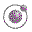The functions generate-leq and the helping function list-index . Lecture 4 - slide 34 : 34Program 1
```;; Generate a less than or equal predicate from the
;; enumeration-order. If p is the generated predicate,
;; (p x y) is true if and only if (selector x) comes before
;; (or at the same position) as (selector y) in the
;; enumeration-order. Thus, (selector x) is assumed to give a
;; value in enumeration-order. Comparison with elements in the
;; enumeration-list is done with eq?
(define (generate-leq enumeration-order selector)
(lambda (x y)
; x and y supposed to be elements in enumeration order
(let ((x-index (list-index (selector x) enumeration-order))
(y-index (list-index (selector y) enumeration-order)))
(<= x-index y-index))))

; A helping function of generate-leq.
; Return the position of e in lst. First is 1
; compare with eq?
; if e is not member of lst return (+ 1 (length lst))
(define (list-index e lst)
(cond ((null? lst) 1)
((eq? (car lst) e) 1)
(else (+ 1 (list-index e (cdr lst))))))```